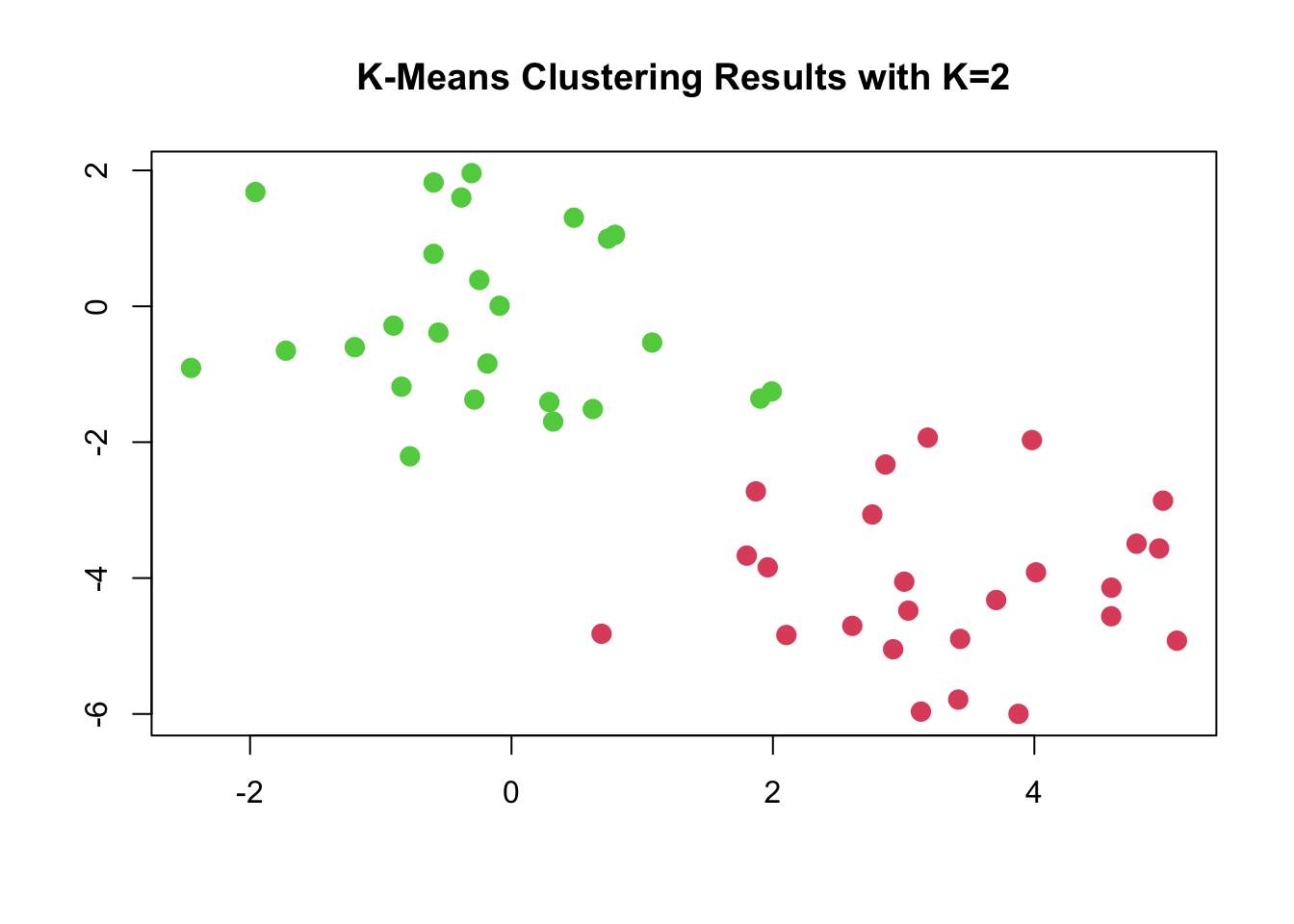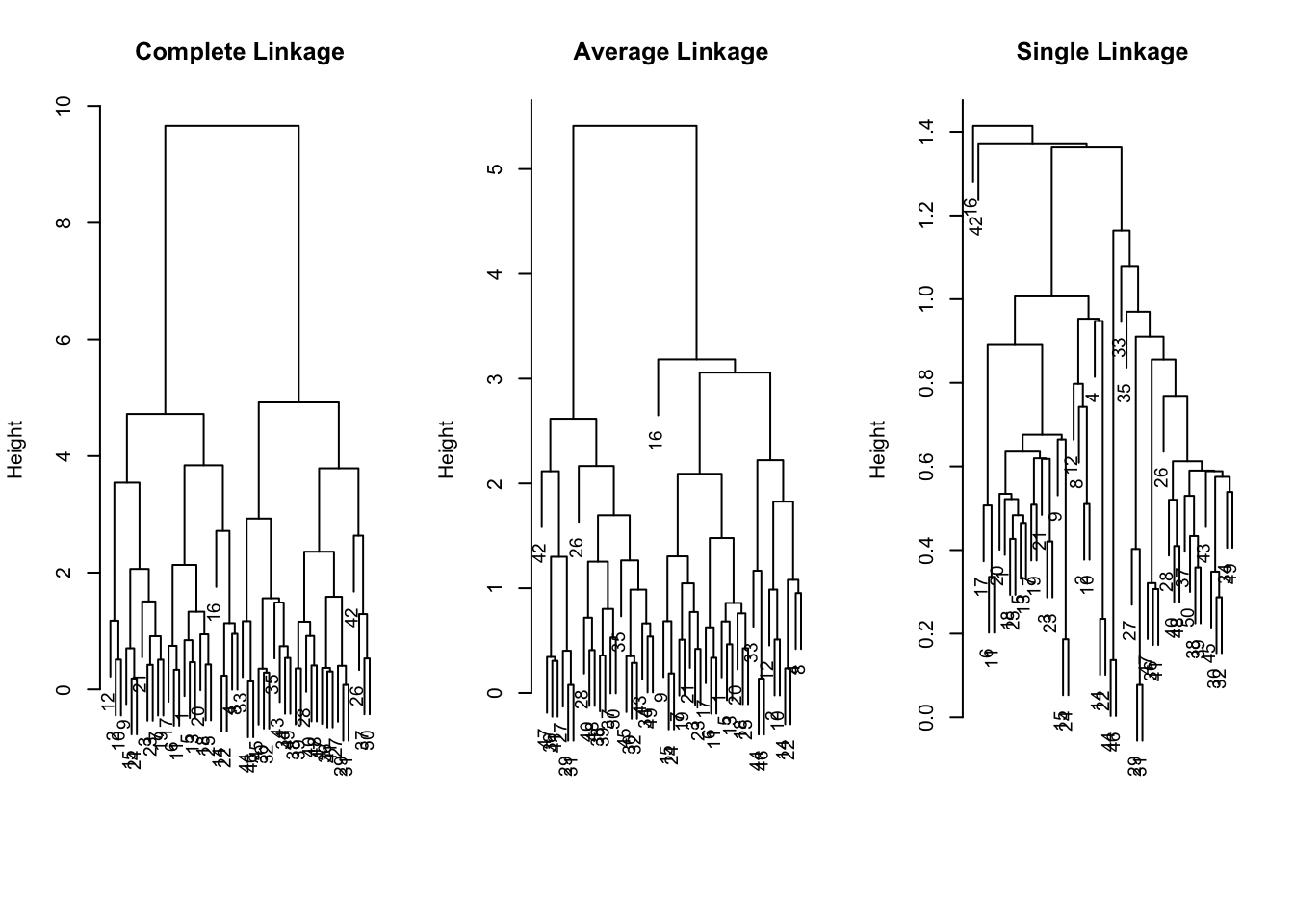p404

# K-Means Clustering

kmeans()

``````set.seed(2)
x=matrix(rnorm(50*2), ncol=2)
x[1:25,1]=x[1:25,1]+3
x[1:25,2]=x[1:25,2]-4``````

## kmeans, k=2

``````km.out=kmeans(x,2,nstart=20)
km.out\$cluster``````
``````##   1 1 1 1 1 1 1 1 1 1 1 1 1 1 1 1 1 1 1 1 1 1 1 1 1 2 2 2 2 2 2 2 2 2 2 2 2 2
##  2 2 2 2 2 2 2 2 2 2 2 2``````

The K-means clustering perfectly separated the observations into two clusters

``plot(x, col=(km.out\$cluster + 1), main="K-Means Clustering Results with K=2", xlab="", ylab="", pch=20, cex=2)``## kmeans, k=3

``````set.seed (4)
km.out=kmeans(x,3,nstart=20)
km.out``````
``````## K-means clustering with 3 clusters of sizes 17, 23, 10
##
## Cluster means:
##         [,1]        [,2]
## 1  3.7789567 -4.56200798
## 2 -0.3820397 -0.08740753
## 3  2.3001545 -2.69622023
##
## Clustering vector:
##   1 3 1 3 1 1 1 3 1 3 1 3 1 3 1 3 1 1 1 1 1 3 1 1 1 2 2 2 2 2 2 2 2 2 2 2 2 2
##  2 2 2 2 2 3 2 3 2 2 2 2
##
## Within cluster sum of squares by cluster:
##  25.74089 52.67700 19.56137
##  (between_SS / total_SS =  79.3 %)
##
## Available components:
##
##  "cluster"      "centers"      "totss"        "withinss"     "tot.withinss"
##  "betweenss"    "size"         "iter"         "ifault"``````

To run the kmeans() function in R with multiple initial cluster assignments, we use the nstart argument. If a value of nstart greater than one is used, then K-means clustering will be performed using multiple random assignments in Step 1 of Algorithm 10.1, and the kmeans() function will report only the best results.

Compare using nstart=1 to nstart=20

``````set.seed(3)
km.out=kmeans(x,3,nstart=1)
km.out\$tot.withinss #  104.3319``````
``##  97.97927``
``````km.out=kmeans(x,3,nstart=20)
km.out\$tot.withinss``````
``##  97.97927``

We strongly recommend always running K-means clustering with a large value of nstart, such as 20 or 50

# Hierarchical Clustering

p406

hclust() function implements hierarchical clustering in R

``hc.complete=hclust(dist(x), method="complete")``

We could just as easily perform hierarchical clustering with average or single linkage instead

``````hc.average=hclust(dist(x), method="average")
hc.single=hclust(dist(x), method="single")``````

## Dendograms

``````par(mfrow=c(1,3))
plot(hc.complete, main="Complete Linkage", xlab="", sub="", cex =.9)
plot(hc.average, main="Average Linkage", xlab="", sub="", cex =.9)
plot(hc.single, main="Single Linkage", xlab="", sub="", cex =.9)``````## Cut Tree

To determine the cluster labels for each observation associated with a given cut of the dendrogram, we can use the cutree() function

``cutree(hc.complete, 2)``
``````##   1 1 1 1 1 1 1 1 1 1 1 1 1 1 1 1 1 1 1 1 1 1 1 1 1 2 2 2 2 2 2 2 2 2 2 2 2 2
##  2 2 2 2 2 2 2 2 2 2 2 2``````
``cutree(hc.average, 2)``
``````##   1 1 1 1 1 1 1 1 1 1 1 1 1 1 1 1 1 1 1 1 1 1 1 1 1 2 2 2 2 2 2 2 1 2 2 2 2 2
##  2 2 2 2 2 1 2 1 2 2 2 2``````
``cutree(hc.single, 2)``
``````##   1 1 1 1 1 1 1 1 1 1 1 1 1 1 1 2 1 1 1 1 1 1 1 1 1 1 1 1 1 1 1 1 1 1 1 1 1 1
##  1 1 1 1 1 1 1 1 1 1 1 1``````
``cutree(hc.single, 4)``
``````##   1 1 1 1 1 1 1 1 1 1 1 1 1 1 1 2 1 1 1 1 1 1 1 1 1 3 3 3 3 3 3 3 3 3 3 3 3 3
##  3 3 3 4 3 3 3 3 3 3 3 3``````

To scale the variables before performing hierarchical clustering of the observations, we use the scale() function.

``````xsc=scale(x)
plot(hclust(dist(xsc), method="complete"), main="Hierarchical Clustering with Scaled Features ")``````